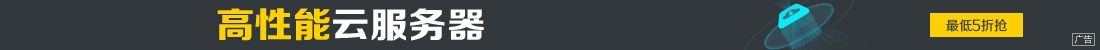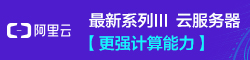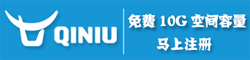# Python进阶篇之字典操作总结

••```>>> d = {'a':3, 'b':2, 'c':6}
>>> min(zip(d.values(), d.keys()))
(2, 'b')```

```>>> d = {'a':3, 'b':2, 'c':6}
>>> min((v ,k) for (k, v) in d.items())
(2, 'b')```

```>>> l = [('a', 1), ('b', 2), ('c', 3)]
>>> {k: v for k, v in l}
{'c': 3, 'b': 2, 'a': 1}```

```d1 = {'a':1, 'b':2, 'c':3, 'd':4}
d2 = {'b':2, 'c':3, 'd':3, 'e':5}```

```>>> dict(d1.items() & d2.items()) # 取交集
{'b': 2, 'c': 3}```

```>>> { k:d1[k] for k in d1.keys() & d2.keys() }
{'b': 2, 'd': 4, 'c': 3}```

```>>> { k:d1[k] for k in d1.keys() - {'c', 'd'} } # - 号的含义是集合的差集操作
{'b': 2, 'a': 1}```

```d1 = {'a':1, 'b':2, 'c':3}
d2 = {'c':4, 'd':5, 'e':6}```

```>>> from collections import ChainMap

>>> chain_dict = ChainMap(d1, d2)
>>> for k, v in chain_dict.items():
print(k, v)
a 1
e 6
d 5
c 3
b 2```

ChainMap 将传入的多个字典连接为一个字典，并返回一个 ChainMap 对象，这个对象的行为就像一个单一的字典，我们可以对其进行取值或者迭代等操作。注意到这里键 c 对应的值为 3，如果传入 ChainMap 的字典含有相同的键，则对应的值为先传入的字典中的值。

```>>> from itertools import chain
>>> for k, v in chain(d1.items(), d2.items()):
print(k, v)

a 1
c 3
b 2
e 6
c 4
d 5```

```>>> d = dict()
>>> d['a'] = 1
>>> d['b'] = 2
>>> d['c'] = 3
>>> for k, v in d.items():
print(k, v)

a 1
c 3
b 2```

```>>> from collections import OrderedDict
>>> ordered_d = OrderedDict()
>>> ordered_d['a'] = 1
>>> ordered_d['b'] = 2
>>> ordered_d['c'] = 3
>>> for k, v in ordered_d.items():
print(k, v)

a 1
b 2
c 3```

OrderedDict 实际通过维护一个双向链表来记录元素添加的顺序，因此其耗费的内存大约为普通字典的两倍。所以在实际使用中需综合考虑各种因素来决定是否使用 OrderedDict 。

```>>> from pprint import pprint
>>> l = [('a', 1), ('a', 2), ('b', 3), ('b', 4), ('c', 5)]
>>> d = {}
>>> for k, v in l:
if k in d:
d[k].append(v)
else:
d[k] = [v]

>>> pprint(d)
{'a': [1, 2], 'b': [3, 4], 'c': }```

```>>> from collections import defaultdict
>>> d = defaultdict(list)
>>> for k, v in l:
d[k].append(v)

>>> pprint(d)
defaultdict(<class 'list'>, {'c': , 'b': [3, 4], 'a': [1, 2]})```

`if else` 的判语句没有了。

defaultdict 是 dict 的一个子类。对 dict 来说，如果 key 不存在，则 dict[key] 取值操作会抛出 KeyError 异常，但是 defaultdict 则会返回一个传入 defaultdict 构造器的类的实例（例如一个列表）或者自定义的缺失值。因此在上例中，对于` d[k].append(v) `，当 k 不存在时，则会先执行 d[k] = [] 并返回这个空列表，继而将 v 加入到列表中。

```>>> from collections import defaultdict
>>> def zero_default():
return 0

>>> d = defaultdict(zero_default)
>>> d['a'] = 1
>>> d['a']
1

>>> d['b']
0

>>> d.keys()
dict_keys(['b', 'a'])
>>>```

```>>> from collections import defaultdict
>>> import json
>>> tree = lambda: defaultdict(tree)
>>> d = tree()
>>> d['a']['b'] = 1
>>> print(json.dumps(d)) # 为了显示的格式更好看
{"a": {"b": 1}}```